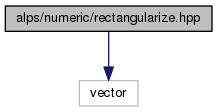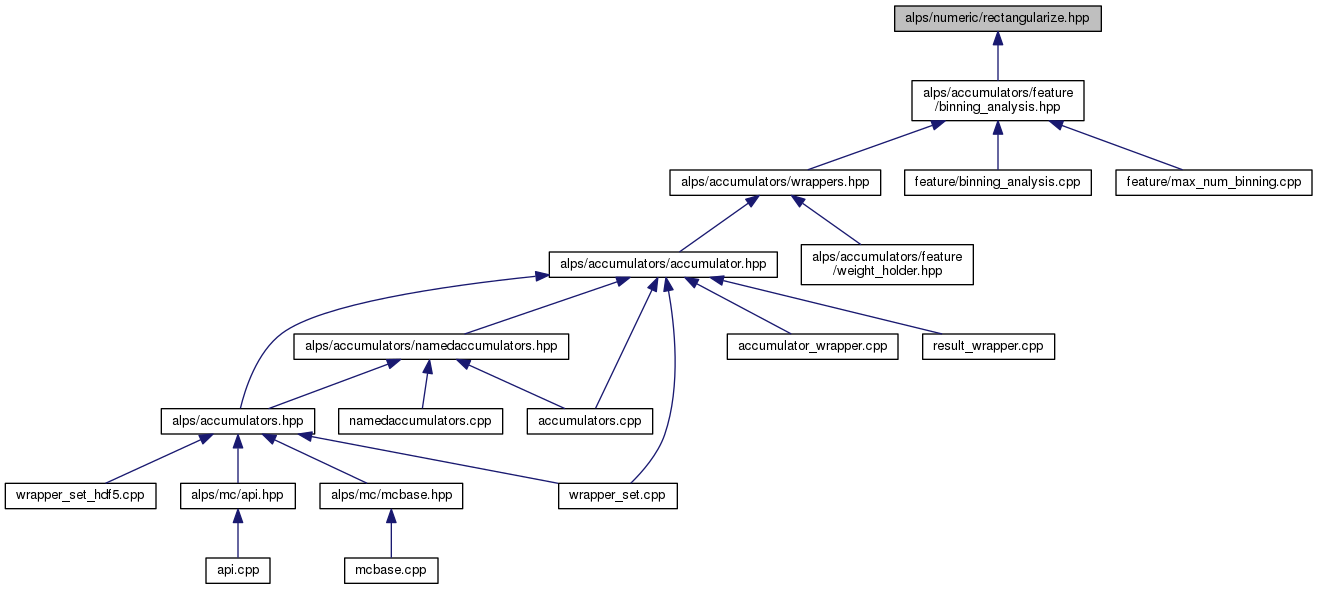ALPSCore reference
rectangularize.hpp File Reference

Defines a function to rectangularize a vector-of-vectors. More...

#include <vector>
Include dependency graph for rectangularize.hpp:This graph shows which files directly or indirectly include this file:Go to the source code of this file.

alps

alps::numeric

## Functions

template<typename T >
void alps::numeric::rectangularize (const T &)
Make sure that vector-of-vectors is a rectangular matrix (generic dummy template) More...

template<typename T >
void alps::numeric::rectangularize (std::vector< std::vector< T > > &vec)
Make sure that vector-of-vectors is a rectangular matrix. More...

## Detailed Description

Defines a function to rectangularize a vector-of-vectors.

Definition in file rectangularize.hpp.